# Trigonometric Ratios of Some Special Angles

#### notes

1) Trignometric Ratios of 45°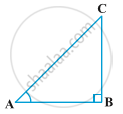In ∆ ABC, right-angled at B, if one angle is 45°, then the other angle is also 45°, i.e., ∠ A = ∠ C = 45°

If two angles are equal then the sides opposite to them are also equal

therefore, BC = AB

Now, Suppose BC = AB = a.

Then by Pythagoras Theorem, "AC"^2 = "AB"^2 + "BC"^2 = a^2 + a^2 = 2a^2,

and, therefore, AC = asqrt2.

Using the definitions of the trigonometric ratios, we have :

sin45°="perpendicular"/ "hypotenuse"= "BC"/"AC"=a/(asqrt2) = 1/sqrt2

cos45°= "base"/ "hypotenuse"= "AB"/"AC"= a/ (asqrt2) = 1/sqrt2

tan45°= "perpendicular"/"base"= "BC"/"AB"= a/a= 1

Also, cosec 45° = 1/(sin45°)= sqrt2,

sec 45° = 1/(cos45°)= sqrt2,

cot 45° = 1/(tan45°) = 1.

2)Trigonometric Ratios of 30° and 60°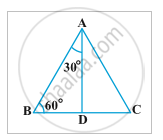Let us now calculate the trigonometric ratios of 30° and 60°. Consider an equilateral triangle ABC. Since each angle in an equilateral triangle is 60°, therefore, ∠ A = ∠ B = ∠ C = 60°.

Draw the perpendicular AD from A to the side BC

AD is the common side in ∆ ABD and ∆ ACD

ABD ≅ ∆ ACD (Angle-Side-Angle rule)

Therefore, BD = DC

ABD is a right triangle, right-angled at D with ∠ BAD = 30° and ∠ ABD = 60°

let us suppose that AB = 2a.

Then, BD =1/2"BC"= a

"AB"^2= "AD"^2+"BD"^2

2a^2= "AD"^2+ a^2

4a^2= "AD"^2+a^2

"AD"^2= 3a^2

"AD"= asqrt3

Now, we have,

sin 30°= "BD"/"AB"= a/(2a)= 1/2,

cos 30°= "AD"/"AB"= (asqrt3)/(2a)= sqrt3/2,

tan30°= "BD"/"AD"= a/(asqrt3)= 1/sqrt3,

Also, cosec  30°= 1/(sin30°)=2,

sec30°= 1/(cos30°)= 2/sqrt3 and

cot30°= 1/(tan30°)= sqrt3

Similarly, sin60°= "AD"/"AB"= (asqrt3)/(2a)= sqrt3/2,

cos60°= 1/2,

tan60°= sqrt3,

cosec60°= 2/sqrt3,

sec60°=2

and cot60°= 1/sqrt3.

3) Trigonometric Ratios of 0°-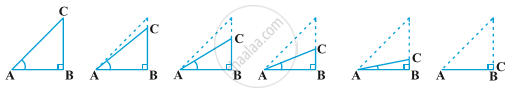Let us see what happens to the trigonometric ratios of angle A, if it is made smaller and smaller in the right triangle ABC, till it becomes zero. As ∠ A gets smaller and smaller, the length of the side BC decreases. The point C gets closer to point B, and finally when ∠ A becomes very close to 0°, AC becomes almost the same as AB.

BC=0 and AC=AB

sin0°= "BC"/"AC"= 0/"AC"= 0,

cos0°= "AB"/"AC"= 1,

tan0°= "BC"/"AB"= 0/"AB"= 0,

cot0°= 1/(tan0°)= not defined,

sec0°= 1/(cos0°)= 1

and cosec0°= 1/(sin0°)= not defined.

4)Trigonometric Ratios of 90°-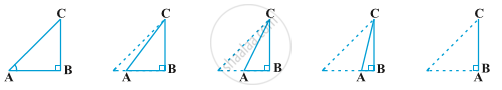Now, let us see what happens to the trigonometric ratios of ∠ A, when it is made larger and larger in ∆ ABC till it becomes 90°. As ∠ A gets larger and larger, ∠ C gets smaller and smaller. Therefore, as in the case above, the length of the side AB goes on decreasing. The point A gets closer to point B. Finally when ∠ A is very close to 90°, ∠ C becomes very close to 0° and the side AC almost coincides with side BC.

AB=0 and AC=BC

sin90°= "BC"/"AC"= 1,

cos90°= "AB"/"AC"= 0/"AC"= 0,

tan90°= "BC"/"AB"= "BC"/0= not defined,

cot90°= "AB"/"BC"= 0/"BC"= 0,

sec90°= "AC"/"AB"= "AC"/0= not defined

and cosec90°= "AC"/"BC"= 1.

Let's sum up this information in a table,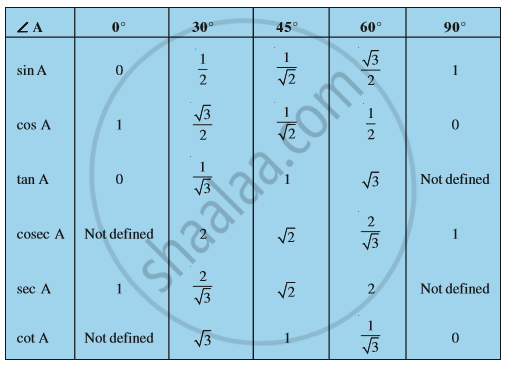If you would like to contribute notes or other learning material, please submit them using the button below.

#### Video Tutorials

We have provided more than 1 series of video tutorials for some topics to help you get a better understanding of the topic.

Series 1

Series 2

### Shaalaa.com

Introduction to Trigonometry part 7 (30 , 45 degree ratio) [00:10:58]
S
0%